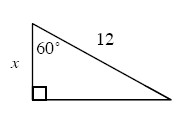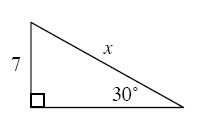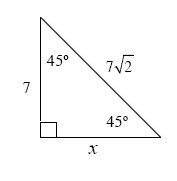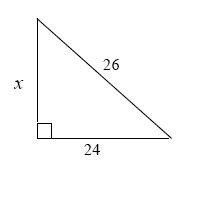### Home > AC > Chapter 14 > Lesson 14.3.1.1 > Problem3-21

3-21.

Find the length of the side labeled $x$ in each triangle below.

1.Look at the angle measures. Does this type of triangle look familiar?

If you cannot solve it that way, refer to problem 1-61 or the Math Notes box in Lesson 1.2.1.

1.$14$

1.Look at the values given. Is it necessary to use the trigonometric identities, or is there an easier way?

1.Use the Pythagorean Theorem.